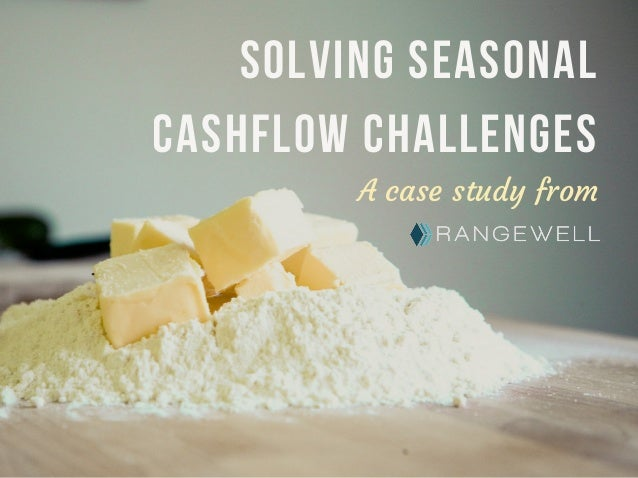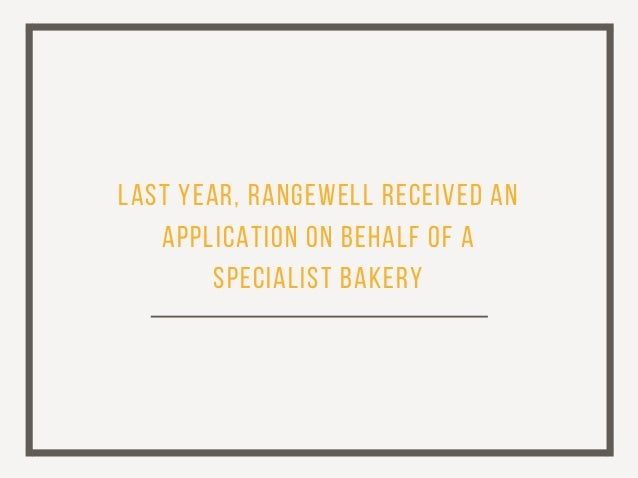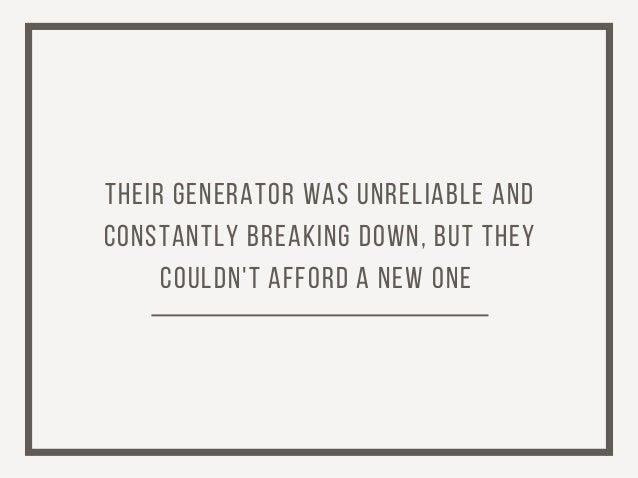Successfully reported this slideshow.Upcoming SlideShare
×

of0

Share

# Solving Seasonal Cashflow Challenges - a Case Study from Rangewell

Seasonal cashflow is a problem for many small businesses, but at Rangewell, it's easy to solve. We used the wide-ranging network of business lenders on our platform to find the perfect business finance solution for this bakery's unique needs. Click through to find out how we did it.

Our platform is the only in-depth and independent map of the complete business finance market in the UK, covering thousands of loan products from over 200 business lenders.
Rangewell helps accountants and their clients to transparently and at no cost find, compare, and apply for every type of business finance product available in the UK.

We're now partners with 2020 Finance, part of the 2020 Innovation group, providing provide accountants with the simplest, quickest and most transparent way to help their clients navigate the business finance market. Find out more at 2020finance.co.uk

See all

### Related Audiobooks

#### Free with a 30 day trial from Scribd

See all
• Be the first to like this

### Solving Seasonal Cashflow Challenges - a Case Study from Rangewell

1. 1. S o l v i n g s e a s o n a l c a s h f l o w c h a l l e n g e s A case study from
2. 2. L a s t ye a r , R a n g e we l l r e c e i v e d a n a p p l i c a t i o n o n b e h a l f o f a s p e c i a l i s t b a k e r y
3. 3. T h e i r g e n e r a t o r wa s u n r e l i a b l e a n d c o n s t a n t l y b r e a k i n g d o wn , b u t t h e y c o u l d n 't a f f o r d a n e w o n e
4. 4. T h e b a k e r y h a d t r i e d t o r a i s e f u n d i n g a l r e a d y, b u t h a d r e a c h e d a d e a d e n d b e c a u s e o f t h e n a t u r e o f t h e i r c a s h f l o w
5. 5. 80% o f t h e b a k e r y's r e v e n u e wa s g e n e r a t e d b e t we e n O c t o b e r a n d M a r c h
6. 6. T h a t m e a n t t h a t t h e y c o u l d n 't k e e p u p r e p a ym e n t s o n s t a n d a r d l o a n s d u r i n g t h e s l o w s u m m e r m o n t h s
7. 7. S o t h e i r a d v i s o r c a m e t o R a n g e we l l
8. 8. a n d we f o u n d a solution
9. 9. W i t h o v e r 200 b u s i n e s s l e n d e r s o n o u r p l a t f o r m , i t wa s n 't h a r d t o f i n d s o m e o n e wh o c o u l d h e l p
10. 10. W i t h o n e o f o u r l e n d e r s , we e n g i n e e r e d a h i r e p u r c h a s e a g r e e m e n t wi t h l o w p a ym e n t s i n J u n e , J u l y, a n d Au g u s t
11. 11. T h i s r e m o v e d t h e s e a s o n a l s t r a i n o n t h e i r c a s h f l o w a n d a l l o we d t h e m t o p u r c h a s e t h e g e n e r a t o r t h e y n e e d e d s o m u c h
12. 12. and that's not all...
13. 13. T h e b a k e r y's a d v i s o r s we r e p l e a s e d t o f i n d o u t t h a t R a n g e we l l k n e w q u i t e a f e w m o r e b u s i n e s s l e n d e r s wh o we r e a b l e t o c o n s t r u c t c a s h f l o w-m a t c h e d a g r e e m e n t s l i k e t h i s
14. 14. S o t h e y'd n e v e r h a v e t o wo r r y a b o u t f i n d i n g f u n d i n g f o r t h e i r c l i e n t a g a i n
15. 15. (a n d we 'd n e v e r h a v e t o wo r r y a b o u t r u n n i n g l o w o n c a k e )
16. 16. W i t h o u r wi d e n e t wo r k o f b u s i n e s s l e n d e r s , R a n g e we l l c a n f i n d f i n a n c e t o s u i t e v e r y b u s i n e s s ' u n i q u e n e e d s .
17. 17. O u r p l a t f o r m h a s t h o u s a n d s o f p r o d u c t s f r o m o v e r 200 b u s i n e s s l e n d e r s , s o yo u c a n b e s u r e yo u h a v e a c c e s s t o t h e wi d e s t r a n g e o f d e a l s f o r yo u r c l i e n t s .
18. 18. I f yo u wa n t t o b e c o m e p a r t o f a s t o r y l i k e t h i s o n e , v i s i t r a n g e we l l .c o m

Total views

657

On Slideshare

0

From embeds

0

Number of embeds

9

4

Shares

0